Analyzing a circuit network and finding out the current or voltage is a tough job. However, analyzing a circuit will be easy if we apply the proper process to reduce complexity. The basic circuit network analyzing techniques are Mesh Current Analysis and Nodal Voltage Analysis.

### Mesh and Nodal analysis

Mesh and nodal analysis have a specific set of rules and limited criteria to get the perfect result out of it. For the working of a circuit, single or multiple voltage or current source or both is required. Determination of Analysis technique is an important step in solving the circuit. And it depends on the number of voltage or current source available in the specific circuit or networks.

Mesh analysis depends on the available voltage source whereas nodal analysis depends on the current source. So, for simpler calculation and to reduce complexity, it is a wiser choice to use mesh analysis where a large number of voltage sources are available. At the same time if the circuit or networks deals with a large number of current sources, then Nodal analysis is the best choice.

But what if a circuit has both voltage and current sources? If a circuit has a larger number of voltage sources and few numbers of current sources, still Mesh analysis is the best choice, but the trick is to change the current sources into an equivalent voltage source.

In this tutorial, we will discuss Mesh analysis and will understand how to use it in a circuit network.

### Mesh Current Method or Analysis

To analyze a network with mesh analysis a certain condition needs to be fulfilled. The mesh analysis is only applicable to planner circuits or networks.

What is a planar circuit?

Planner circuit is a simple circuit or network that can be drawn on a plane surface where no crossover is happening. When the circuit needs a crossover then it is a nonplanar circuit.

Below image shows a planar circuit. It is simple and no crossover is present.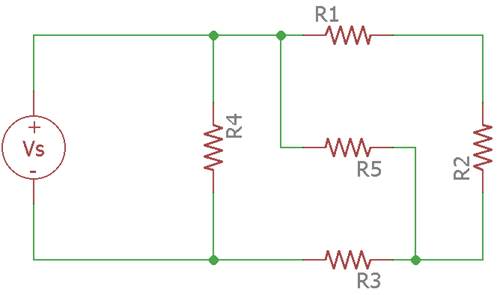Now below circuit is a nonplanar circuit. The circuit cannot be simplified as there is crossover in the circuit.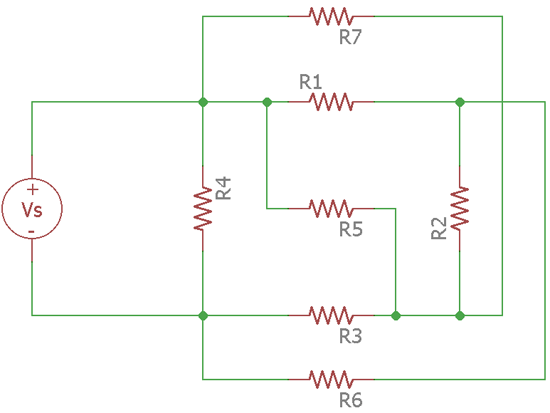Mesh analysis cannot be done in the nonplanar circuit and, it can only be done in the planar circuit. To apply the Mesh Analysis, few simple steps are required to get the end result.

1. The first step is to identify whether it is a planar circuit or nonplanar circuit.
2. If it is a planar circuit then it needs to be simplified without any crossover.
3. Identifying the Meshes.
4. Identifying the voltage source.
5. Finding out the current circulating path
6. Applying Kirchoff's law in proper places.

Let's see how Mesh Analysis can be a helpful process for circuit level analysis.

### Finding current in Circuit using Mesh Current Method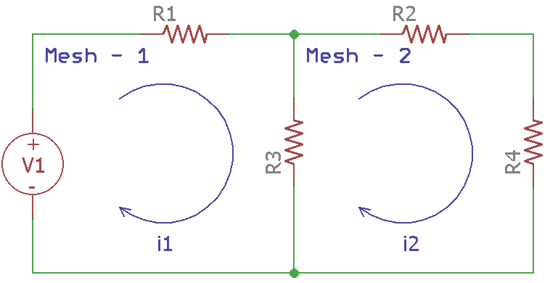The above circuit contains two meshes. It is a simple planner circuit where 4 resistors are present. The first mesh is created using R1 and R3 resistors and the second mesh is created using R2, R4, and R3.

Two different value of current is flowing through each mesh. The Voltage source is V1. The circulating current in each mesh can be easily identified using the mesh equation.

For the first mesh, V1, R1, and R3 are connected in series. Therefore, they both share the same current which is denoted as the blue circulating identifier named as i1. For the second mesh, the exact same thing is happening, R2, R4, and R3 share the same current which is also denoted as a blue circulating line, denoted as i2.

There is a special case for the R3. R3 is a common resistor between two meshes. That means two different currents of two different meshes is flowing through the resistor R3. What will be the current of R3? It is the difference between the two mesh or loop current. So, the current flowing through the resistor R3 is i1 – i2.

Let's consider first mesh-

By applying Kirchhoff’s voltage law, The Voltage of V1 is equal to the voltage difference of R1 and R3.

Now what is the voltage of R1 and R3? For this case, Ohms law will be very helpful. As per Ohms law Voltage = Current x Resistance.

So, for R1 the voltage is i1 x R1and for the resistor R3, it will be (i1 – i2) x R3

Therefore, as per the Kirchoff’s voltage law,

`V1 = i1R1 + R3(i1 – i2)………..[Equation: 1]`

For the second mesh, there is no voltage source present like the V1 in the first mesh. In such case, as per the Kirchhoff’s voltage law, in a closed loop series circuit network path, the potential differences of all resistors are equal to 0.

So, by applying the same Ohms law and Kirchhoff’s law,

`R3(i1 – i2)) + i2R2 + i2R4 = 0)………..[Equation: 2]`

By solving Equation 1 and Equation 2, the value of i1 and i2 can be identified. Now we will see two practical examples to solve the circuit loops.

### Solving Two Meshes using Mesh Current Analysis

What will be the mesh current of the following circuit?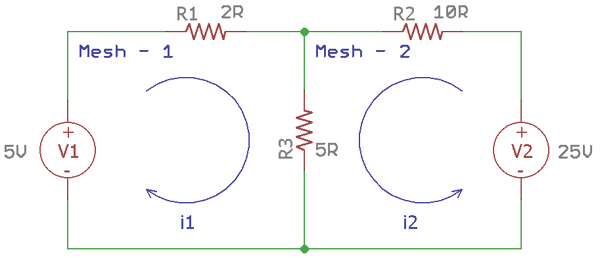The above circuit network is slightly different than the previous example. In the previous example, the circuit had a single voltage source V1, but for this circuit network, there is two different voltage source is present, V1 and V2. There are two meshes in the circuitry.

For Mesh-1, V1, R1, and R3 are connected in series. So, the same current is flowing through the three components which are i1.

By using the Ohms law, the voltage of each component is-

```V1 = 5V
VR1 = i1 x 2 = 2i1```

For the R3, two loop currents are flowing through it as this is a shared component between two meshes. As there is two different voltage source for different meshes, the current through the resistor R3 is i1+i2.

So, the voltage at

`VR3 = (i1+i2) x 5 = 5(i1+i2)`

As per the Kirchhoff's law,

```V1 = 2i1 + 5(i1+i2)
5 = 7i1 + 5i2……. (Equation:1)```

Now in Mesh-2, V2, R2, and R3 are connected in series. So, the same current is flowing through the three components which is i2.

By using the Ohms law, the voltage of each component are-

```V1 = 25V
VR2 = i2 x 10 = 10i2
VR3 = (i1 + i2) x 5 =5(i1 + i2)```

As per the Kirchhoff's law,

```V2 = 10i2 + 5(i1+i2)
25 = 5i1+15i2
5 = i1+ 3i2….. (Equation: 2)
```

So, Here are the two equations, 5 = 7i1+ 5i2 and5 = i1+ 3i2.

By solving this two equation we get,

```i1 = .625A
i2 = 1.875A```

The circuit further simulated in spice tool to evaluate the result.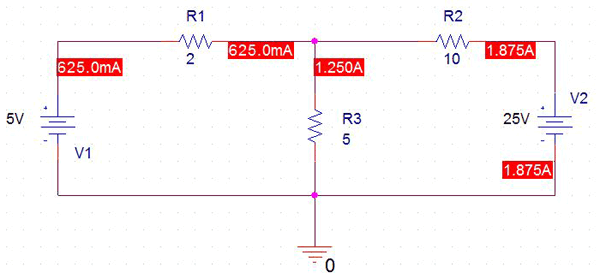The exact same circuit is replicated in Orcad Pspice and we get the same result

### Solving Three Meshes using Mesh Current Analysis

Here is another classic Mesh analysis example

Let’s consider the below circuit network. By using Mesh analysis, we will calculate the three currents in three meshes.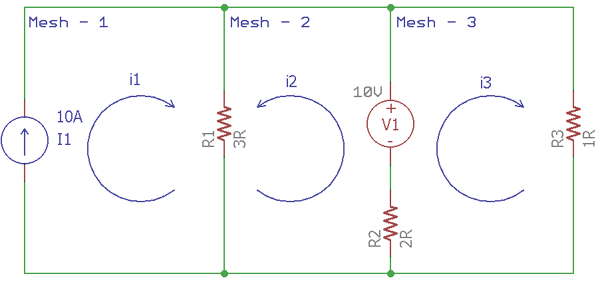The above circuit network has three meshes. An additional current source is also available.

To solve the circuit network in the mesh analysis process, Mesh-1 is ignored as the i1, a ten Ampere current source is outside of the circuit network.

In Mesh-2, V1, R1, and R2 are connected in series. So, the same current is flowing through the three components which is i2.

By using the Ohms law, the voltage of each component are-

`V1 = 10V`

For R1 and R2, two loop currents are flowing through each Resistor. R1 is a shared component between two meshes, 1 and 2. So the current flowing through the resistor R1 is i2 – i2. Same as the R1, The current through the resistor R2 is i2 – i3.

Therefore, the voltage across the resistor R1

`VR1 = (i2 – i1) x 3 = 3 (i2 – i1)`

And for the resistor R2

`VR2 = 2 x (i2 – i3) = 2(i2 – i3)`

As per the Kirchhoff's law,

`3(i2 – i1) + 2(i2 – i3) + 10 = 0 or -3i1 + 5i2 = -10…. (Equation: 1)`

So, the value of  i1 is already known which is 10A.

By providing the ivalue, Equation:2 can be formed.

```-3i1 + 5i2 – 2i3 = -10
-30 + 5i2 – 2i3 = -10
5i2 – 2i3 = 20…. (Equation: 2)```

In Mesh-3, V1, R3, and R2 are connected in series. So, the same current is flowing through the three components which is i3.

By using the Ohms law, the voltage of each component is-

```V1 = 10V
VR2 = 2 (i3 – i2)
VR3 = 1 x i3 = i3```

As per the Kirchhoff's law,

```i3 + 2 (i3 – i2) = 10
or, -2i2 + 3i3 = 10….[Equation:3]
```

Therefore, Here are two equation, 5i2 – 2i3 = 20 and -2i2 + 3i3 = 10. By solving these two equations, i2 = 7.27A and i3 = 8.18A.

The Mesh analysis simulation in pspice showed the exact same result as calculated.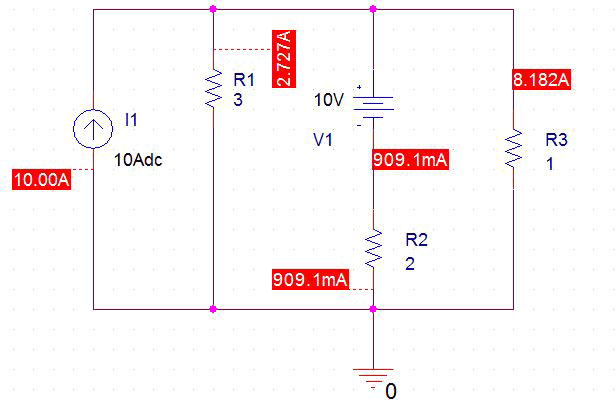This is how current can be calculated in loops and meshes using Mesh Current Analysis.